cheatsheet最后更新于：2020年6月30日

# Supervised Learning cheatsheet

## Introduction to Supervised Learning

Given a set of data points $\{x^{(1)}, ..., x^{(m)}\}$ associated to a set of outcomes $\{y^{(1)}, ..., y^{(m)}\}$, we want to build a classifier that learns how to predict $y$ from $x$.

• Type of prediction: The different types of predictive models are summed up in the table below:
Regression Classifier
Outcome Continuous Class
Examples Linear regression Logistic regression, SVM, Naive Bayes
• Type of model: The different models are summed up in the table below:
Discriminative model Generative model
Goal Directly estimate $P(x|y)$ Estimate $P(x|y)$ to then deduce $P(y|x)$
What's learned Decision boundary Probability distributions of the data
Illustration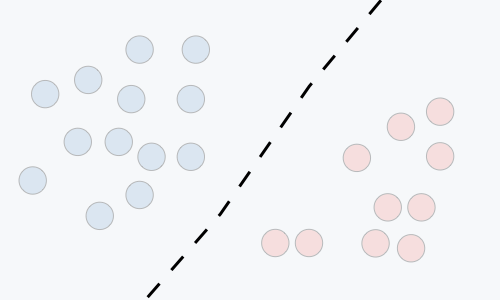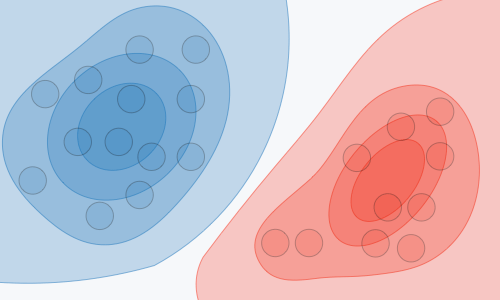Examples Regressions, SVMs GDA, Naive Bayes

## Notations and general concepts

• Hypothesis: The hypothesis is noted $h_\theta$ and is the model that we choose. For a given input data $x^{(i)}$ the model prediction output is $h_\theta(x^{(i)})$.
• Loss function: A loss function is a function $L:(z,y)\in\mathbb{R}\times Y\longmapsto L(z,y)\in\mathbb{R}$ that takes as inputs the predicted value $z$ corresponding to the real data value $y$ and outputs how different they are. The common loss functions are summed up in the table below:
Least squared error Logistic loss Hinge loss Cross-entropy
$\quad \displaystyle\frac{1}{2}(y-z)^2 \quad$ $\displaystyle\log(1+\exp(-yz))$ $\max(0,1-yz)$ $(y-1)\log(1-z)$
$-y\log(z)$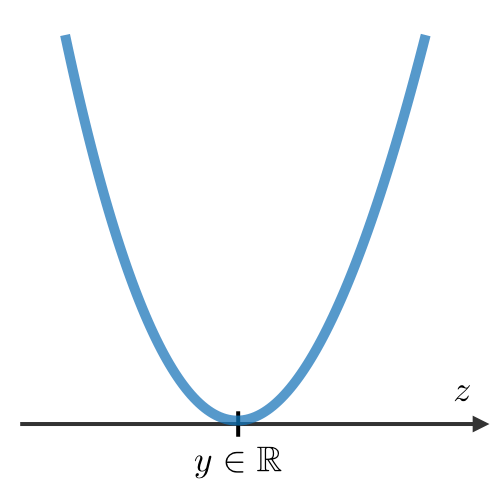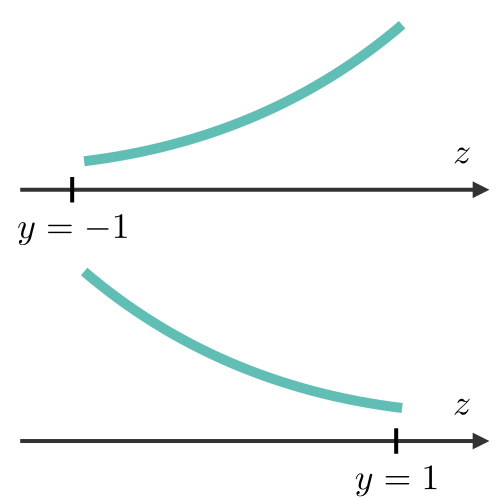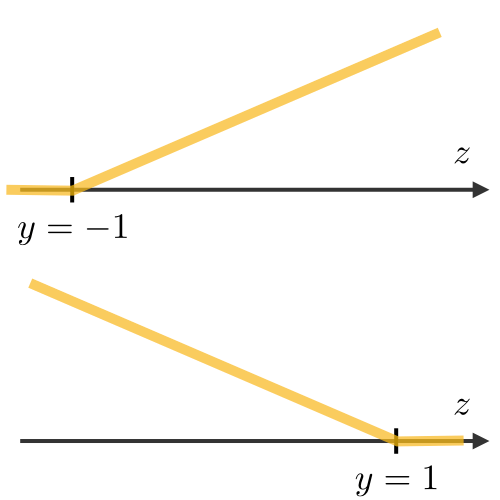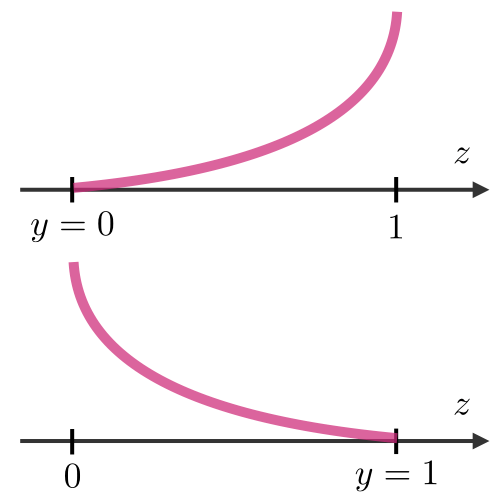Linear regression Logistic regression SVM Neural
• Cost function: The cost function $J$ is commonly used to assess the performance of a model, and is defined with the loss function $L$ as follows:

$\boxed{J(\theta)=\sum_{i=1}^mL(h_\theta(x^{(i)}), y^{(i)})}$

• Gradient descent: By noting $\alpha\in\mathbb{R}$ the learning rate, the update rule for gradient descent is expressed with the learning rate and the cost function $J$ as follows:

$\boxed{\theta\longleftarrow\theta-\alpha\nabla J(\theta)}$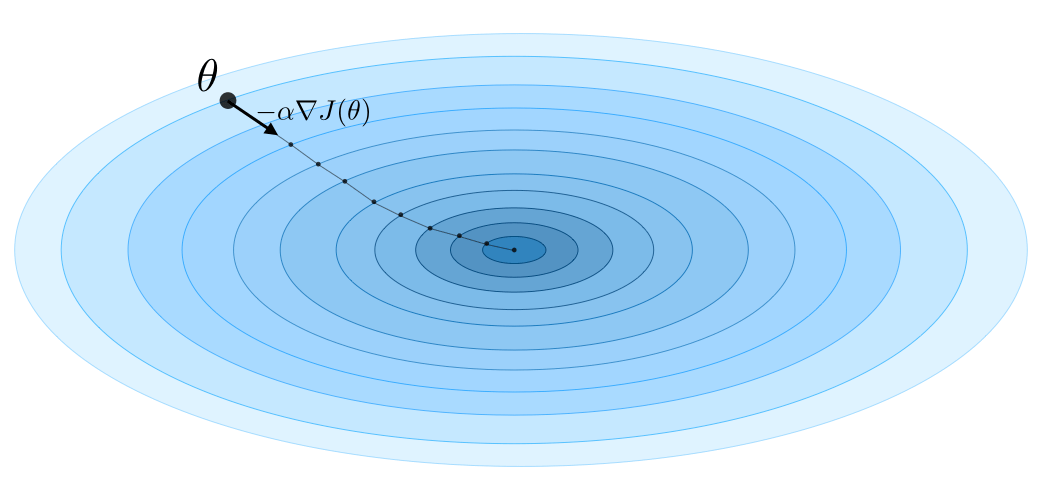Remark: Stochastic gradient descent (SGD) is updating the parameter based on each training example, and batch gradient descent is on a batch of training examples.

• Likelihood: The likelihood of a model $L(\theta)$ given parameters $\theta$ is used to find the optimal parameters $\theta$ through maximizing the likelihood. In practice, we use the log-likelihood $\ell(\theta)=\log(L(\theta))$ which is easier to optimize. We have:

$\boxed{\theta^{\textrm{opt}}=\underset{\theta}{\textrm{arg max }}L(\theta)}$

• Newton's algorithm: The Newton's algorithm is a numerical method that finds $\theta$ such that $\ell'(\theta)=0$. Its update rule is as follows:

$\boxed{\theta\leftarrow\theta-\frac{\ell'(\theta)}{\ell^{\prime\prime}(\theta)}}$

Remark: the multidimensional generalization, also known as the Newton-Raphson method, has the following update rule: $\theta\leftarrow\theta-\left(\nabla_\theta^2\ell(\theta)\right)^{-1}\nabla_\theta\ell(\theta)$

## Linear models

### Linear regression

We assume here that $y|x;\theta\sim\mathcal{N}(\mu,\sigma^2)$

• Normal equations: By noting $X$ the design matrix, the value of $\theta$ that minimizes the cost function is a closed-form solution such that:

$\boxed{\theta=(X^TX)^{-1}X^Ty}$

• LMS algorithm: By noting $\alpha$ the learning rate, the update rule of the Least Mean Squares (LMS) algorithm for a training set of $m$ data points, which is also known as the Widrow-Hoff learning rule, is as follows:

$\boxed{\forall j,\quad \theta_j \leftarrow \theta_j+\alpha\sum_{i=1}^m\left[y^{(i)}-h_\theta(x^{(i)})\right]x_j^{(i)}}$

Remark: the update rule is a particular case of the gradient ascent.

• LWR: a variant of linear regression that weights each training example in its cost function by $w^{(i)}(x)$, which is defined with parameter $\tau\in\mathbb{R}$ as:

$\boxed{w^{(i)}(x)=\exp\left(-\frac{(x^{(i)}-x)^2}{2\tau^2}\right)}$

### Classific ation and logistic regression

• Sigmoid function: The sigmoid function $g$, also known as the logistic function, is defined as follows:

$\forall z\in\mathbb{R},\quad\boxed{g(z)=\frac{1}{1+e^{-z}}\in]0,1[}$

• Softmax regression: A softmax regression, also called a multiclass logistic regression, is used to generalize logistic regression when there are more than 2 outcome classes. By convention, we set $\theta_K=0$, which makes the Bernoulli parameter $\phi_i$ of each class $i$ equal to:

$\boxed{\displaystyle\phi_i=\frac{\exp(\theta_i^Tx)}{\displaystyle\sum_{j=1}^K\exp(\theta_j^Tx)}}$

### Generalized Linear Models

• Exponential family: A class of distributions is said to be in the exponential family if it can be written in terms of a natural parameter, also called the canonical parameter or link function, $\eta$, a sufficient statistic $T(y)$ and a log-partition function $a(\eta)$ as follows:

$\boxed{p(y;\eta)=b(y)\exp(\eta T(y)-a(\eta))}$

Remark: we will often have $T(y)=y$. Also, $\exp(-a(\eta))$ can be seen as a normalization parameter that will make sure that the probabilities sum to one.

Here are the most common exponential distributions summed up in the following table:

Distribution $\eta$ $T(y)$ $a(\eta)$ $b(y)$
Bernoulli $\log\left(\frac{\phi}{1-\phi}\right)$ $y$ $\log(1+\exp(\eta))$ $1$
Gaussian $\mu$ $y$ $\frac{\eta^2}{2}$ $\frac{1}{\sqrt{2\pi}}\exp\left(-\frac{y^2}{2}\right)$
Poisson $\log(\lambda)$ $y$ $e^{\eta}$ $\frac{1}{y!}$
Geometric $\log(1-\phi)$ $y$ $\log\left(\frac{e^\eta}{1-e^\eta}\right)$ $1$
• Assumptions of GLMs: Generalized Linear Models (GLM) aim at predicting a random variable $y$ as a function of $x\in\mathbb{R}^{n+1}$ and rely on the following 3 assumptions:
• $(1)\quad\boxed{y|x;\theta\sim\textrm{ExpFamily}(\eta)}$
• $(2)\quad\boxed{h_\theta(x)=E[y|x;\theta]}$
• $(3)\quad\boxed{\eta=\theta^Tx}$

Remark: ordinary least squares and logistic regression are special cases of generalized linear models.

## Support Vector Machines

The goal of support vector machines is to find the line that maximizes the minimum distance to the line.

• Optimal margin classifier: The optimal margin classifier $h$ is such that:

$\boxed{h(x)=\textrm{sign}(w^Tx-b)}$

where $(w, b)\in\mathbb{R}^n\times\mathbb{R}$ is the solution of the following optimization problem: $\boxed{\min\frac{1}{2}\|w\|^2}\quad\quad\textrm{such that }\quad \boxed{y^{(i)}(w^Tx^{(i)}-b)\geqslant1}$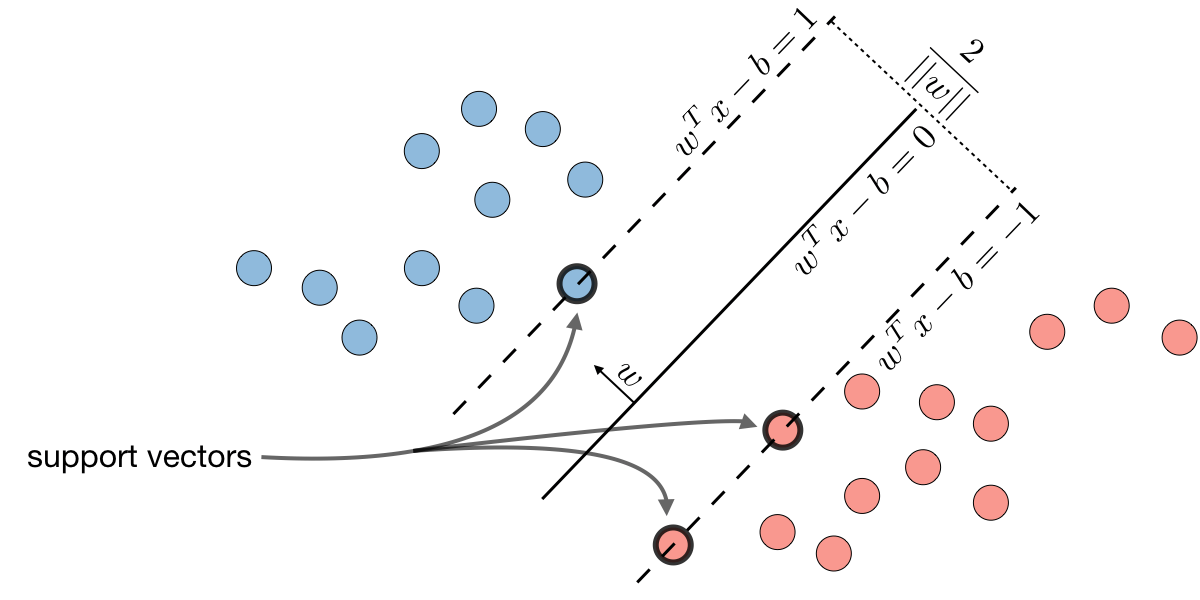Remark: the line is defined as $\boxed{w^Tx-b=0}$.

• Hinge loss: The hinge loss is used in the setting of SVMs and is defined as follows:

$\boxed{L(z,y)=[1-yz]_+=\max(0,1-yz)}$

• Kernel: Given a feature mapping $\phi$, we define the kernel $K$ to be defined as:

$\boxed{K(x,z)=\phi(x)^T\phi(z)}$

In practice, the kernel $K$ defined by $K(x,z)=\exp\left(-\frac{\|x-z\|^2}{2\sigma^2}\right)$ is called the Gaussian kernel and is commonly used.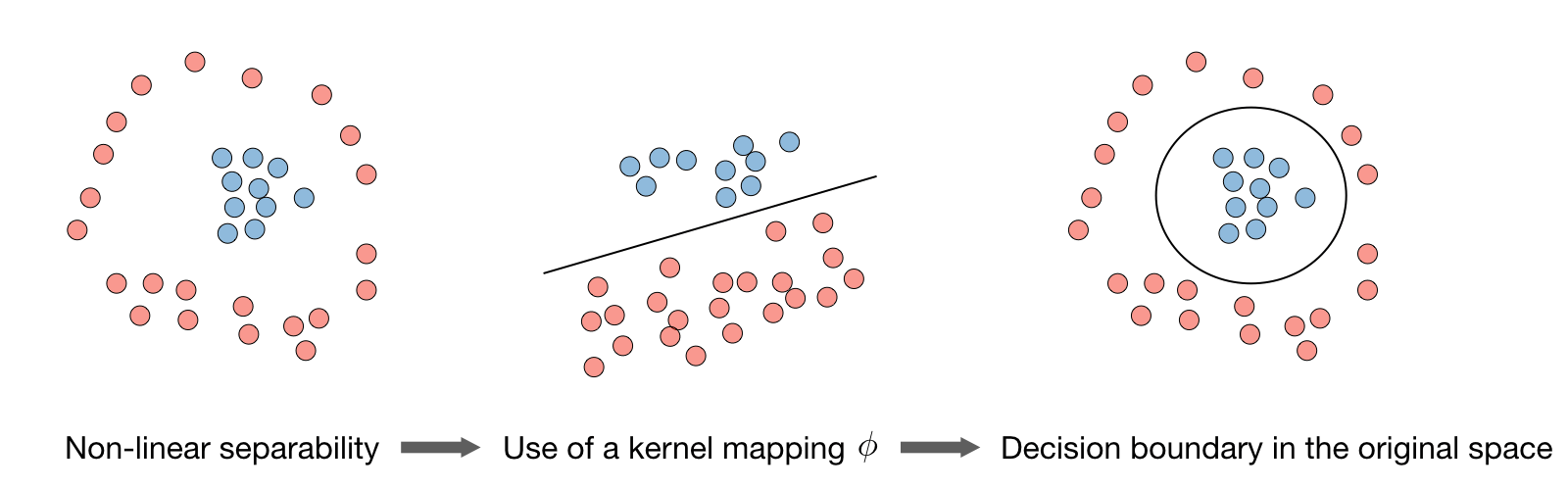Remark: we say that we use the "kernel trick" to compute the cost function using the kernel because we actually don't need to know the explicit mapping $\phi$, which is often very complicated. Instead, only the values $K(x,z)$ are needed.

• Lagrangian: We define the Lagrangian $\mathcal{L}(w,b)$ as follows:

$\boxed{\mathcal{L}(w,b)=f(w)+\sum_{i=1}^l\beta_ih_i(w)}$

Remark: the coefficients $\beta_i$ are called the Lagrange multipliers.

## Generative Learning

A generative model first tries to learn how the data is generated by estimating $P(x|y)$, which we can then use to estimate $P(y|x)$ by using Bayes' rule.

### Gaussian Discriminant Analysis

• Setting:The Gaussian Discriminant Analysis assumes that $y$ and $x|y=0$ and $x|y=1$ are such that:
• $(1)\quad\boxed{y\sim\textrm{Bernoulli}(\phi)}$
• $(2)\quad\boxed{x|y=0\sim\mathcal{N}(\mu_0,\Sigma)}$
• $(3)\quad\boxed{x|y=1\sim\mathcal{N}(\mu_1,\Sigma)}$
• Estimation: The following table sums up the estimates that we find when maximizing the likelihood:
$\widehat{\phi}$ $\displaystyle\frac{1}{m}\sum_{i=1}^m1_{(y^{(i)}=1)}$
$\widehat{\mu_j}\quad{\small(j=0,1)}$ $\displaystyle\frac{\sum_{i=1}^m1_{(y^{(i)}=j)}x^{(i)}}{\sum_{i=1}^m1_{(y^{(i)}=j)}}$
$\widehat{\Sigma}$ $\displaystyle\frac{1}{m}\sum_{i=1}^m(x^{(i)}-\mu_{y^{(i)}})(x^{(i)}-\mu_{y^{(i)}})^T$

### Naive Bayes

• Assumption: The Naive Bayes model supposes that the features of each data point are all independent:

$\boxed{P(x|y)=P(x_1,x_2,...|y)=P(x_1|y)P(x_2|y)...=\prod_{i=1}^nP(x_i|y)}$

• Solutions: Maximizing the log-likelihood gives the following solutions, with $k\in\{0,1\},l\in[\![1,L]\!]$

\boxed{ \begin{align} P(y=k)&=\frac{1}{m}\times\#\{j|y^{(j)}=k\}\\ P(x_i=l|y=k)&=\frac{\#\{j|y^{(j)}=k\textrm{ and }x_i^{(j)}=l\}}{\#\{j|y^{(j)}=k\}} \end{align} }

Remark: Naive Bayes is widely used for text classification and spam detection.

## Tree-based and ensemble methods

These methods can be used for both regression and classification problems.

• CART: Classification and Regression Trees (CART), commonly known as decision trees, can be represented as binary trees. They have the advantage to be very interpretable.

• Random forest: It is a tree-based technique that uses a high number of decision trees built out of randomly selected sets of features. Contrary to the simple decision tree, it is highly uninterpretable but its generally good performance makes it a popular algorithm.

Remark: random forests are a type of ensemble methods.

• Boosting: The idea of boosting methods is to combine several weak learners to form a stronger one. The main ones are summed up in the table below:
• High weights are put on errors to improve at the next boosting step
• Weak learners trained on remaining errors

## Other non-parametric approaches

• $k$-nearest neighbors: The $k$-nearest neighbors algorithm, commonly known as $k$-NN, is a non-parametric approach where the response of a data point is determined by the nature of its $k$ neighbors from the training set. It can be used in both classification and regression settings.

Remark: The higher the parameter $k$, the higher the bias, and the lower the parameter $k$, the higher the variance.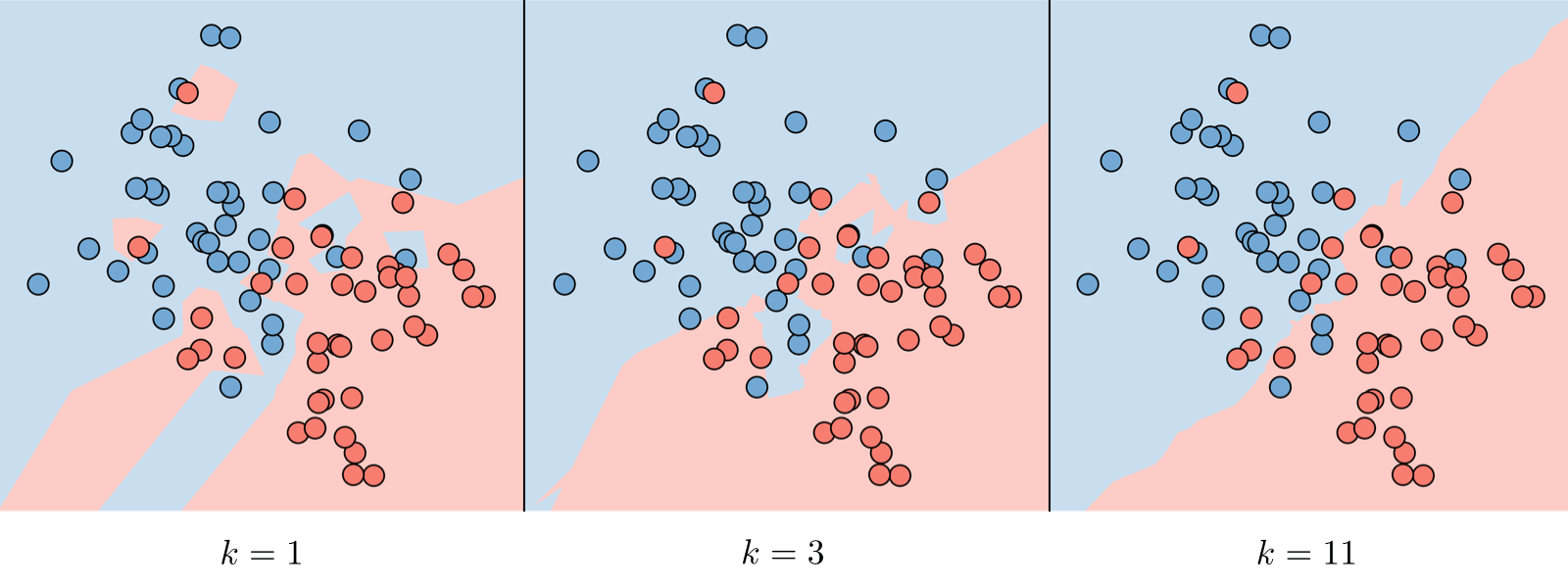## Learning Theory

• Union boundLet $A_1, ..., A_k$ be $k$ events. We have:

$\boxed{P(A_1\cup ...\cup A_k)\leqslant P(A_1)+...+P(A_k)}$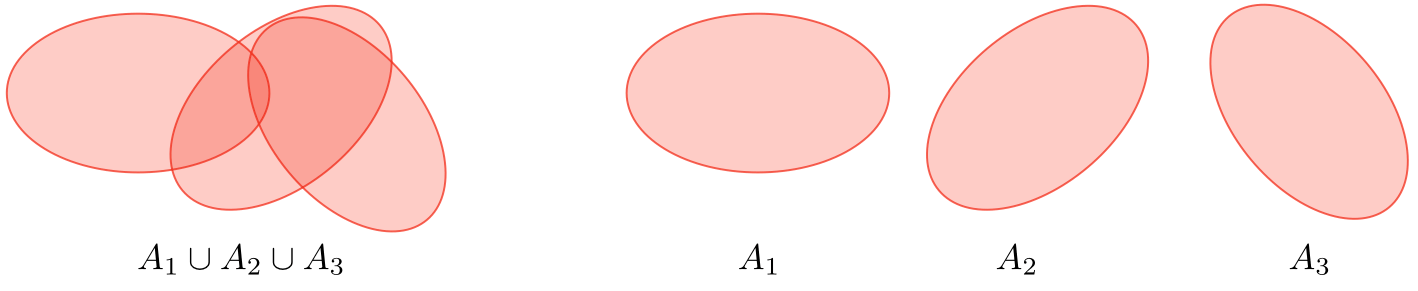• Hoeffding inequality: Let $Z_1, .., Z_m$ be $m$ iid variables drawn from a Bernoulli distribution of parameter $\phi$. Let $\widehat{\phi}$ be their sample mean and $\gamma>0$ fixed. We have:

$\boxed{P(|\phi-\widehat{\phi}|>\gamma)\leqslant2\exp(-2\gamma^2m)}$

Remark: this inequality is also known as the Chernoff bound.

• Training error: For a given classifier $h$, we define the training error $\widehat{\epsilon}(h)$, also known as the empirical risk or empirical error, to be as follows:

$\boxed{\widehat{\epsilon}(h)=\frac{1}{m}\sum_{i=1}^m1_{(h(x^{(i)})\neq y^{(i)})}}$

• Probably Approximately Correct (PAC): PAC is a framework under which numerous results on learning theory were proved, and has the following set of assumptions:
• the training and testing sets follow the same distribution
• the training examples are drawn independently
• Shattering: Given a set $S=\{x^{(1)},...,x^{(d)}\}$, and a set of classifiers $\mathcal{H}$, we say that $\mathcal{H}$ shatters $S$ if for any set of labels $\{y^{(1)}, ..., y^{(d)}\}$, we have:

$\boxed{\exists h\in\mathcal{H}, \quad \forall i\in[\![1,d]\!],\quad h(x^{(i)})=y^{(i)}}$

• Upper bound theorem: Let $\mathcal{H}$ be a finite hypothesis class such that $|\mathcal{H}|=k$ and let $\delta$ and the sample size $m$ be fixed. Then, with probability of at least $1-\delta$, we have:

$\boxed{\epsilon(\widehat{h})\leqslant\left(\min_{h\in\mathcal{H}}\epsilon(h)\right)+2\sqrt{\frac{1}{2m}\log\left(\frac{2k}{\delta}\right)}}$

• VC dimension: The Vapnik-Chervonenkis (VC) dimension of a given infinite hypothesis class $\mathcal{H}$, noted $\textrm{VC}(\mathcal{H})$ is the size of the largest set that is shattered by $\mathcal{H}$.

Remark: the VC dimension of ${\small\mathcal{H}=\{\textrm{set of linear classifiers in 2 dimensions}\}}$ is 3.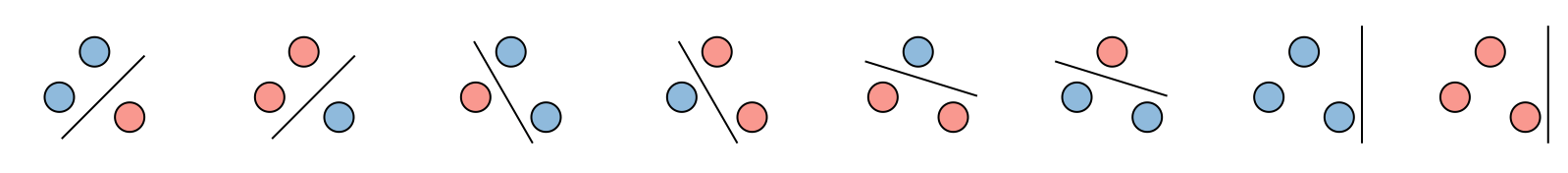• Theorem (Vapnik): Let $\mathcal{H}$ be given, with $\textrm{VC}(\mathcal{H})=d$ and $m$ the number of training examples. With probability at least $1-\delta$, we have:

$\boxed{\epsilon(\widehat{h})\leqslant \left(\min_{h\in\mathcal{H}}\epsilon(h)\right) + O\left(\sqrt{\frac{d}{m}\log\left(\frac{m}{d}\right)+\frac{1}{m}\log\left(\frac{1}{\delta}\right)}\right)}$